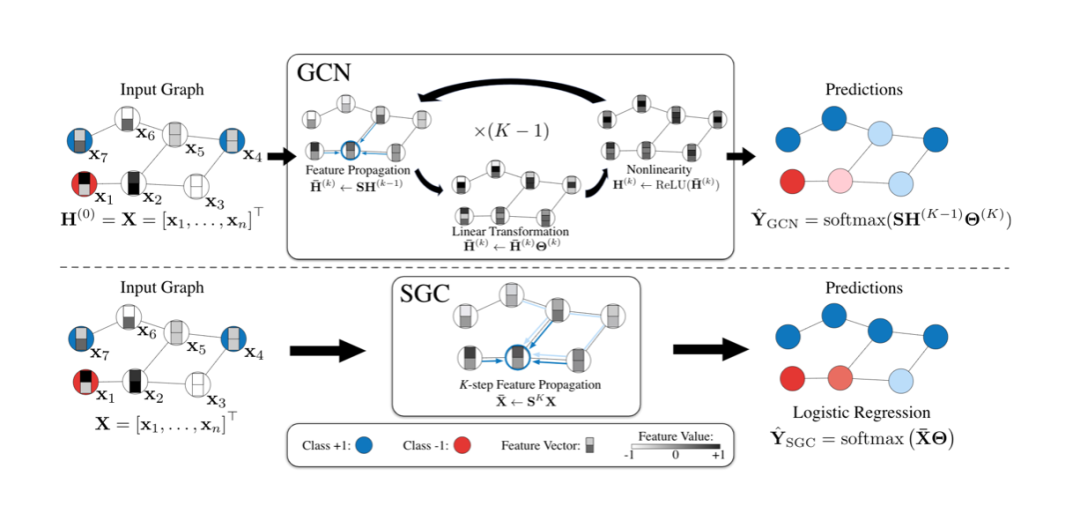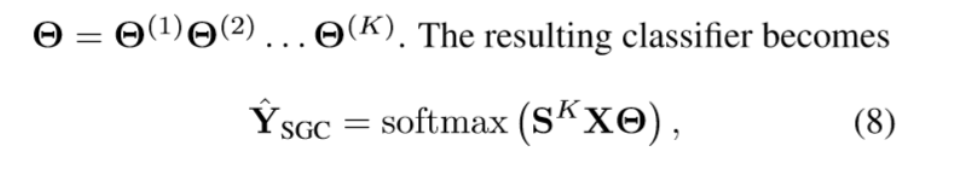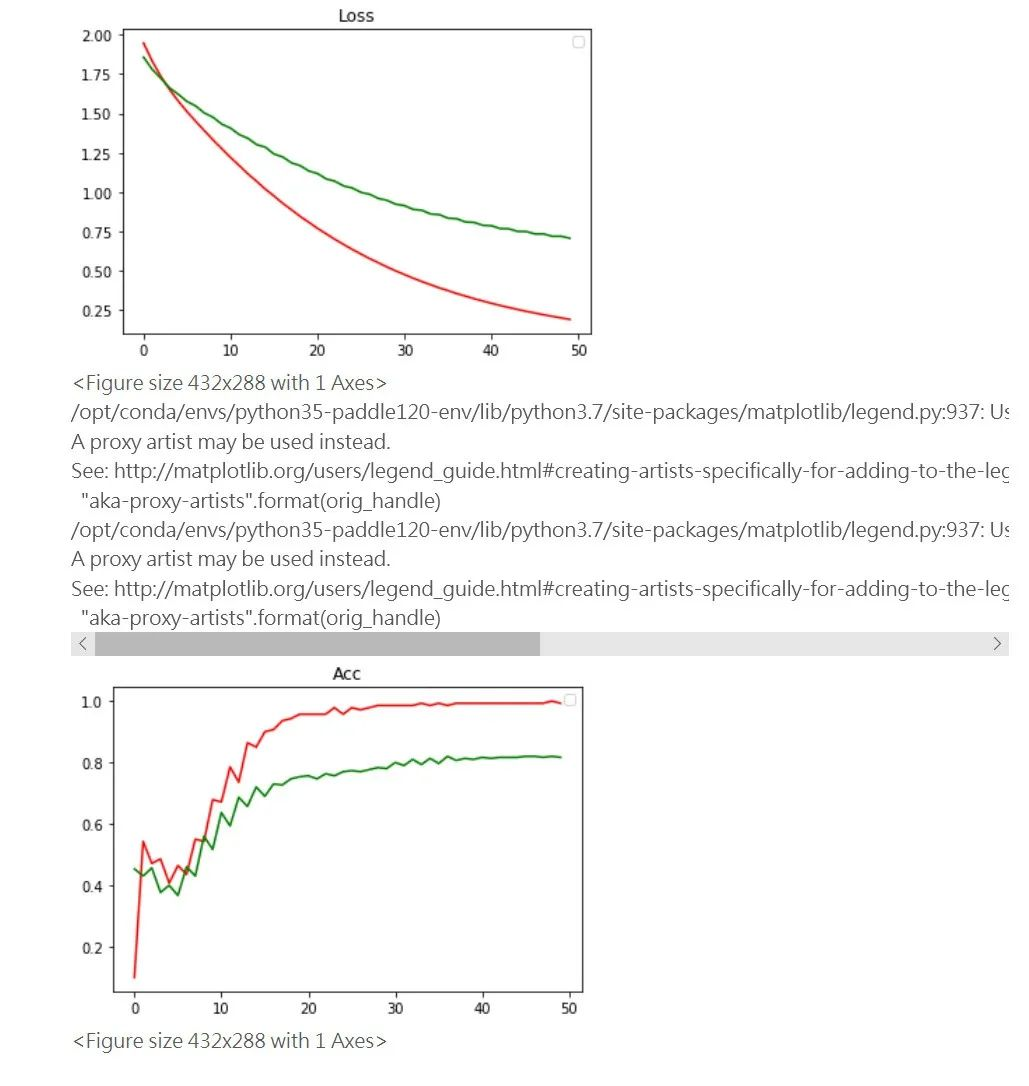# 基于飞桨复现ICML顶会模型SGC，可实现超快速网络收敛

10/15 16:45SGC-Simplifying graph convolutional network 成为了最合适的替代品，在当今大图计算仍然没有一个最优且快的model的时候，在效果上退而求其次是不可避免的。SGC在训练效果上和原始GCN持平，但是在速度上有这不可比拟的优势，而且空间复杂度极小，具有在大图上训练的潜力。

```下载安装命令

## CPU版本安装命令

## GPU版本安装命令SGC最大的创新在于省去了激活函数，从而使得多个参数合成一个，减小了时间复杂度和空间复杂度。## 首先在AI Studio上安装PGL库函数和cython：

```
```
1.
`!pip install pgl  `
2.
`!pip install cython  `
3.

```
```
1.
`import scipy.sparse as sp  `
2.

3.
`degree=dataset.graph.indegree()  `
4.
`norm = np.zeros_like(degree, dtype="float32")  `
5.
`norm[degree > 0] = np.power(degree[degree > 0],-1.0)  `
6.
`dataset.graph.node_feat["norm"] = np.expand_dims(norm, -1)  `
7.
`def row_normalize(mx):  `
8.
`    """Row-normalize sparse matrix"""  `
9.
`    rowsum = np.array(mx.sum(1))  `
10.
`    r_inv = np.power(rowsum, -1).flatten()  `
11.
`    r_inv[np.isinf(r_inv)] = 0.  `
12.
`    r_mat_inv = sp.diags(r_inv)  `
13.
`    mx = r_mat_inv.dot(mx)  `
14.
`    return mx  `
15.
`def aug_normalized_adjacency(adj):  `
16.
`    adj = adj + sp.eye(adj.shape)  `
17.
`    adj = sp.coo_matrix(adj)  `
18.
`    row_sum = np.array(adj.sum(1))  `
19.
`    d_inv_sqrt = np.power(row_sum, -0.5).flatten()  `
20.
`    d_inv_sqrt[np.isinf(d_inv_sqrt)] = 0.  `
21.
`    d_mat_inv_sqrt = sp.diags(d_inv_sqrt)  `
22.
`    return d_mat_inv_sqrt.dot(adj).dot(d_mat_inv_sqrt).tocoo()  `
23.```
```
1.
`def pre_sgc(dataset,feature,norm=None):  `
2.
`   """ `
3.
`   Implementation of Simplifying graph convolutional networks (SGC) `
4.
`   This is an implementation of the paper SEMI-SUPERVISED CLASSIFICATION `
5.
`   WITH Simplifying graph convolutional networks (https://arxiv.org/abs/1902.07153). `
6.
`   Args: `
7.
`       gw: Graph wrapper object (:code:`StaticGraphWrapper` or :code:`GraphWrapper`) `
8.
`       feature: A tensor with shape (num_nodes, feature_size). `
9.
`       output: The output size for sgc. `
10.
`       activation: The activation for the output `
11.
`       name: Gcn layer names. `
12.
`       norm: If :code:`norm` is not None, then the feature will be normalized. Norm must `
13.
`             be tensor with shape (num_nodes,) and dtype float32. `
14.
`   Return: `
15.
`       A tensor with shape (num_nodes, hidden_size) `
16.
`   """  `
17.
`   num_nodes=np.shape(feature)  `
18.
`   adj=np.zeros((num_nodes,num_nodes))  `
19.
`   #print(np.shape(dataset.graph.edges))  `
20.
`   for u in range(len(dataset.graph.edges)):  `
21.
`       adj[dataset.graph.edges[u],dataset.graph.edges[u]]=adj[dataset.graph.edges[u],dataset.graph.edges[u]]=1  `
22.
`   feature=dataset.graph.node_feat['words']  `
23.
`   feature=row_normalize(feature)  `
24.
`   if norm==True:  `
25.
`       adj=aug_normalized_adjacency(adj)  `
26.
`   for i in range(args.degree):  `
27.
`       feature=adj.dot(feature)  `
28.
`   return feature  `
29.https://arxiv.org/pdf/1902.07153.pdf

```下载安装命令

## CPU版本安装命令

## GPU版本安装命令
```

0
0 收藏

### 作者的其它热门文章0 评论
0 收藏
0